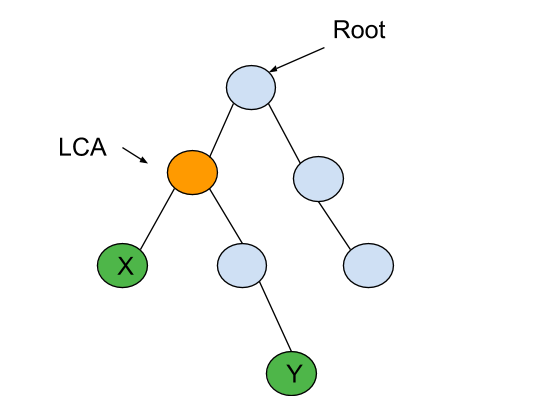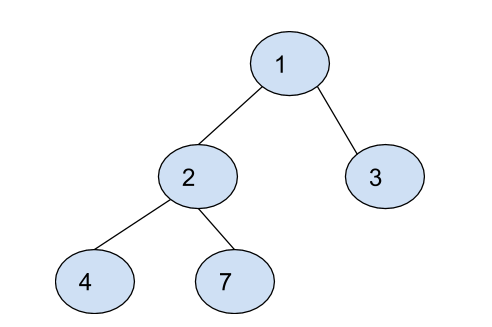Problem title
Difficulty
Avg time to solve

Common Digit Longest Subsequence
Moderate
31 mins
Look-And-Say Sequence
Easy
15 mins
Day of the Week
Easy
10 mins
Next Greater Element
Easy
10 mins
K Most Frequent Words
Moderate
36 mins
Search In Infinite Sorted 0-1 Array
Easy
10 mins
Minimum Depth Of Binary Tree
Moderate
20 mins
Best Time to Buy and Sell
Moderate
20 mins
Stack using queue
Moderate
25 mins
Deepest Leaves Sum
Easy
15 mins8

# LCA Of Binary Tree

Difficulty: MEDIUM
Avg. time to solve
10 min
Success Rate
90%

Problem Statement

#### The LCA of ‘X’ and ‘Y’ in the binary tree is the shared ancestor of ‘X’ and ‘Y’ that is located farthest from the root.

##### Note :
``````You may assume that given ‘X’ and ‘Y’ definitely exist in the given binary tree.
``````
##### For example :
``````For the given binary tree
````````````LCA of ‘X’ and ‘Y’ is highlighted in yellow colour.
``````
##### Input Format :
``````The first line contains an integer 'T' which denotes the number of test cases to be run. Then the test cases follows

The first line of each test case contains elements of the tree in the level order form. The line consists of values of nodes separated by a single space. In case a node is null, we take -1 in its place.

The second line of each test case contains two integers ‘X’ and ‘Y’ denoting the two nodes of the binary tree.

For example, the input for the tree depicted in the below image would be :
````````````1
2 3
4 -1 5 6
-1 7 -1 -1 -1 -1
-1 -1
``````

#### Explanation :

``````Level 1 :
The root node of the tree is 1

Level 2 :
Left child of 1 = 2
Right child of 1 = 3

Level 3 :
Left child of 2 = 4
Right child of 2 = null (-1)
Left child of 3 = 5
Right child of 3 = 6

Level 4 :
Left child of 4 = null (-1)
Right child of 4 = 7
Left child of 5 = null (-1)
Right child of 5 = null (-1)
Left child of 6 = null (-1)
Right child of 6 = null (-1)

Level 5 :
Left child of 7 = null (-1)
Right child of 7 = null (-1)

The first not-null node(of the previous level) is treated as the parent of the first two nodes of the current level. The second not-null node (of the previous level) is treated as the parent node for the next two nodes of the current level and so on.
The input ends when all nodes at the last level are null(-1).
``````
##### Note :
``````The above format was just to provide clarity on how the input is formed for a given tree.
The sequence will be put together in a single line separated by a single space. Hence, for the above-depicted tree, the input will be given as:

1 2 3 4 -1 5 6 -1 7 -1 -1 -1 -1 -1 -1
``````
##### Output Format :
``````For each test case, print an integer denoting the LCA of the given binary tree nodes.

For each test case, print the output in a separate line.
``````
##### Note :
``````You do not need to print anything, it has already been taken care of. Just implement the given function.
``````
##### Constraints :
``````1 <= 'T' <= 100
0 <= 'N' <= 3000
0 <= 'DATA' <= 10^4

Where 'DATA' is a node value of the binary tree.

Time Limit: 1 sec
``````
##### Sample Input 1 :
``````3
1 2 3 4 7 -1 -1 -1 -1 -1 -1
4 7
1 2 3 -1 -1 -1 -1
2 3
4 3 -1 -1 -1
4 3
``````
##### Sample Output 1 :
``````2
1
4
``````
##### Explanation of Sample Input 1 :
``````The tree of the first test case is shown below.
````````````For the first test case, 2 is the common ancestor of 4 and 7, which is farthest from the root node. So, the LCA is 2.

For the second test case, 1 is the only common ancestor of nodes 2 and 3.

For the third test case, 4 is the only common ancestor of nodes 4 and 3.
``````
##### Sample Input 2 :
``````2
2 4 -1 5 -1 -1 -1
4 5
1 4 2 -1 -1 -1 3 -1 -1
4 3
``````
##### Sample Output 2 :
``````4
1
``````
##### Explanation of Sample Input 2 :
``````For the first test case, 4 is the only common ancestor of nodes 4 and 5.

For the second test case, 1 is the only common ancestor of nodes 4 and 3.
``````Console